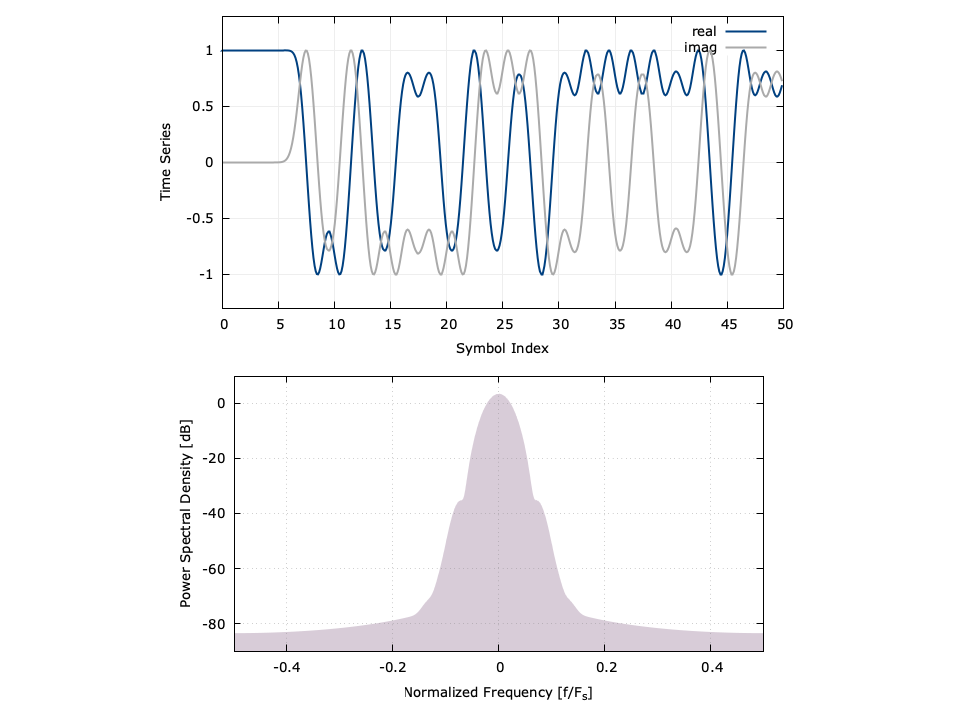# Gauss Minimum-Shift Keying Modulator/Demodulator (gmskmodem)

The two objects gmksmod and gmskdem implement the Gauss minimum-shift keying (GMSK) modem in liquid . Notice that unlike the linear modem objects, the GMSK modulator and demodulator are split into separate objects.

• gmskmod_create(k,m,BT) creates and returns an gmskmod object with $$k$$ samples/symbol, a delay of $$m$$ symbols, and a bandwidth-time product (excess bandwidth factor) $$BT$$ .
• gmskmod_destroy(q) destroys an gmskmod object, freeing all internally-allocated memory.
• gmskmod_reset(q) clears the internal state of the gmskmod object.
• gmskmod_print(q) prints the internal state of the gmskmod object.
• gmskmod_modulate(q,s,*y) modulates a symbol $$s \in \{0,1\}$$ , storing the output in $$k$$ -element array $$\vec{y}$$ .

Demodulation is performed by differentiating the instantaneous received frequency and running the resulting time-varying phase through a matched filter. By design, the GMSK transmit filter imparts inter-symbol interference (by nature of the pulse shape). To mitigate symbol errors, the receive filter is initially designed to remove as much ISI as possible (see [section-filter-firdes_gmsk] for a discussion on GMSK transmit and receive filter designs in liquid ). Internally, the GMSK demodulator takes care of timing recovery using an LMS equalizer (see [section-equalization-eqlms] ). The GMSK demodulator has a similar interface to the modulator:

• gmskdem_create(k,m,BT) creates and returns an gmskdem object with $$k$$ samples/symbol, a delay of $$m$$ symbols, and a bandwidth-time product (excess bandwidth factor) $$BT$$ .
• gmskdem_destroy(q) destroys an gmskdem object, freeing all internally-allocated memory.
• gmskdem_reset(q) clears the internal state of the gmskdem object.
• gmskdem_print(q) prints the internal state of the gmskdem object.
• gmskdem_demodulate(q,*y,*s) demodulates the $$k$$ -element array $$\vec{y}$$ , storing the output symbol ( 0 or 1 ) in the de-referenced pointer $$s$$ .
• gmskdem_set_eq_bw(q,w) sets the bandwidth (learning rate) of the internal LMS equalizer to $$w$$ where $$w \in [0,0.5]$$ .

Listed below is an example to interfacing with the gmskmod and gmskdem modulator/demodulator objects.


#include <liquid/liquid.h>

int main() {
// options
unsigned int k  =    10;    // filter samples/symbol
unsigned int m  =     7;    // filter delay (symbols)
float        BT = 0.25f;    // bandwidth-time product

// create modulator/demodulator objects
gmskmod mod   = gmskmod_create(k, m, BT);
gmskdem demod = gmskdem_create(k, m, BT);

unsigned int  i;
unsigned int  sym_in;       // input data symbol
float complex x[k];         // modulated samples
unsigned int  sym_out;      // demodulated data symbol

{
// generate random symbol {0,1}
sym_in = rand() % 2;

// modulate
gmskmod_modulate(mod, sym_in, x);

// demodulate
gmskdem_demodulate(demod, x, &sym_out);
}

// destroy modem objects
gmskmod_destroy(mod);
gmskdem_destroy(demod);
}


Here is a time series and spectral estimate of the resulting signal:Figure [fig-gmskmodem-psd]. GMSK modem time series for first 50 symbols and power spectral density with an excess bandwidth factor BT=0.25